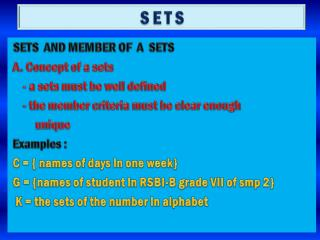DownloadDownload PresentationS E T S

# S E T S

Télécharger la présentation## S E T S

- - - - - - - - - - - - - - - - - - - - - - - - - - - E N D - - - - - - - - - - - - - - - - - - - - - - - - - - -
##### Presentation Transcript

1. S E T S SETS AND MEMBER OF A SETS A. Concept of a sets - a sets must be well defined - the member criteria must be clear enough unique Examples : C = { names of days in one week} G = {names of student in RSBI-B grade VII of smp 2} K = the sets of the number in alphabet

2. B. Elements of a sets Є to express element of a sets  to express not element of a sets Examples : GivenP = { 4, 5, 7, 8, 9 } 4 є P 3  P 7 є P 6  P

3. EXPRESSING SETS 1. by sets in word / by Description P = { even whole numbers less than 15 } Q = { odd whole numbers between 4 and 16} 2. by the roster way / by listing of P = { 2, 4, 6, 8, 10, 12, 14 } Q = { 5, 7, 9, 11, 13, 15 } 3. by the set-builder notation P = { x|x 15, x  G } Q = { x| 4  x  16, x  J }

4. CARDINALITY OF SETS Examples : Given 1. A = { 3, 6, 9, 12, 15 } 2. B = { R S B I } 3. C = { Prime numbers between 10 and 25 } 4. D = Sets of natural numbers less than 8 thus we can determine from those sets sets of A have 6 element or n(A) = 6 sets of B have 4 element or n(B) = 4 sets of C have 5 element or n(C) = 5 sets of D have 7 element or n(D) = 7

5. THE VENN DIAGRAM A. UNIVERSAL SET Universal set is a set containing all elements of the set being talking about and also called the discussing universe. The symbols of universal set is U

6. suppose A = { 1, 2, 3, 4, 5, 6,… 10 } B = { 2, 5, 7, 8 } C = { 1, 3, 6, 7, 9 } D = { 3, 4, 7, 10, 15, 16 } because set A contains all of set B and C, so set A can be said as “ a universal set “ of set B and C. But because 15 and 16 of set D are included in set A, so set A is not a universal set of set D.

7. VENN DIAGRAM Examples : U = { 1, 2, 3, 4,…10 } P = { 2, 4, 7, 9 } venn diagram of them U P 1. 3. 2. 4. 7. 9. 5. 6. 8. 10.

8. SUBSETS AND EMPTY SETS Examples : 1. Given A = { 2, 4 } 2  A 4  A and But { 2 }  A { 3 }  A { 4 }  A { 3, 4 }  A { 2, 4 }  A { } =   A

9. 3. If P = { a, b, c } how many subsets of set P ? Answer { a } { b } { c } { a, b } { a, c } { b, c } { a, b, c } 

10. 4. how many subsets if set Q = { 1, 2, 3, 4 }

11. U INTERSECTION Examples : A B given venn diagram 2. 5. 7. 4. 6. 9. 1. 8. 10. U = {1, 2, 3, 4, 5,…10} A = { 2, 4, 5, 6, 8} B = { 5, 6, 7, 9 } and A  B = { 5, 6 }

12. 3 U 2. 7. 9. 5. 1. 8. A B • From venn diagram Find : a. Set of A b. Set of B c. A  B so if B  A then A  B = B 3 4 6 10

13. Given : U = { 1, 2 , 3 , 4, 5,…10 } A = { 2, 4, 5, 7, 9 } B = { 1, 3, 5, 6, 9 } find : a. make venn diagram b. mention A  B

14. 4. Let U =set of natural number less then 20 A = set of prime number less then 15 B = set of natural number between 2 and 11 C = set of even whole number between 1 and 10 a. draw the venn diagram b. Find A  B d. A  C c. Find B  C e. A  B  C

15. USING THE VENN DIAGRAM TO SOLVE PROBLEMS Examples : 1. from 40 of student, 24 student like music 19 student like sport 8 student like both ( music and sport ) based on the above data a. draw the venn diagram of data b. how many student do not like all

16. 5 U music sport Answer : 16 8 11

17. From 60 student 30 like english 27 like mathematics 25 like science 15 like english and mathematics 13 like mathematics and science 11 like english and science 9 like all a. draw the venn diagram b. determine the number of student do not like the three lessons

18. U english Answer : mathematics 6 13 8 9 2 4 8 10 science